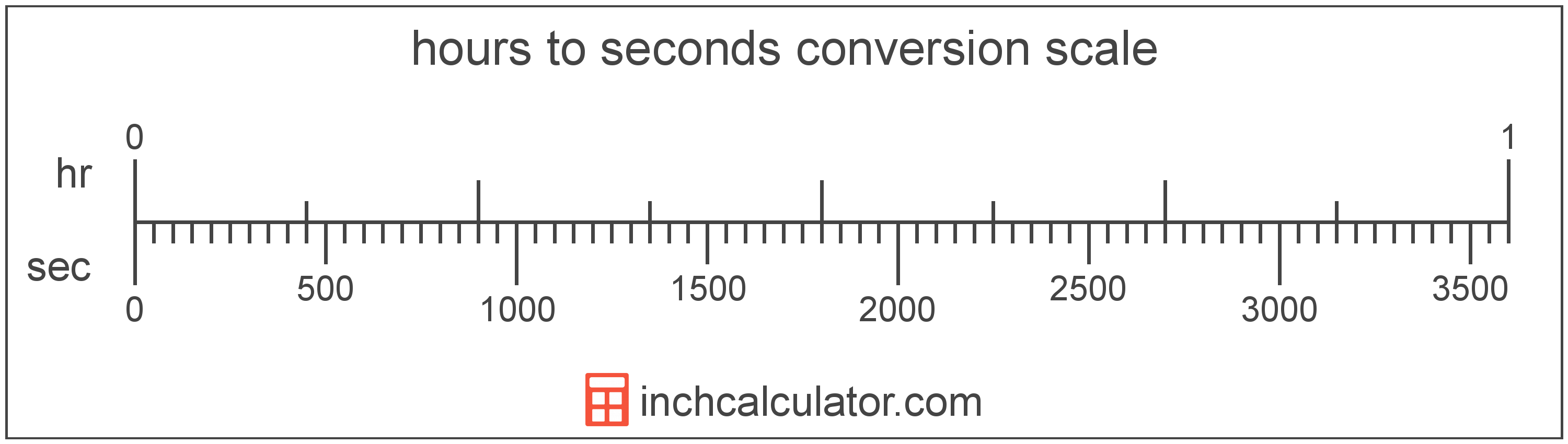# Hours to Seconds Converter

Enter the time in hours below to get the value converted to seconds.

Results in Seconds:1 hr = 3,600 s

Do you want to convert seconds to hours?

## How to Convert Hours to Seconds

To convert a measurement in hours to a measurement in seconds, multiply the time by the following conversion ratio: 3,600 seconds/hour.

Since one hour is equal to 3,600 seconds, you can use this simple formula to convert:

seconds = hours × 3,600

The time in seconds is equal to the time in hours multiplied by 3,600.

For example, here's how to convert 5 hours to seconds using the formula above.
seconds = (5 hr × 3,600) = 18,000 s### How Many Seconds Are in an Hour?

There are 3,600 seconds in an hour, which is why we use this value in the formula above.

1 hr = 3,600 s

## What is an Hour?

The hour is a period of time equal to 1/24 of a day or 60 minutes.

The hour is an SI accepted unit for time for use with the metric system. Hours can be abbreviated as hr (plural hrs); for example, 1 hour can be written as 1 hr, and 2 hours can be written as 2 hrs.

## What is a Second?

The second has historically been defined as 1/60 of a minute or 1/86,400 of a day. According to the SI definition, the second is the duration of 9,192,631,770 oscillations of the frequency of light emitted from the atomic transition between the two hyperfine levels of the unperturbed ground state of the caesium 133 atom.

The second is the SI base unit for time in the metric system. Seconds can be abbreviated as s, and are also sometimes abbreviated as sec. For example, 1 second can be written as 1 s or 1 sec.

## Hour to Second Conversion Table

Table showing various hour measurements converted to seconds.
Hours Seconds
0.001 hr 3.6 s
0.002 hr 7.2 s
0.003 hr 10.8 s
0.004 hr 14.4 s
0.005 hr 18 s
0.006 hr 21.6 s
0.007 hr 25.2 s
0.008 hr 28.8 s
0.009 hr 32.4 s
0.01 hr 36 s
0.02 hr 72 s
0.03 hr 108 s
0.04 hr 144 s
0.05 hr 180 s
0.06 hr 216 s
0.07 hr 252 s
0.08 hr 288 s
0.09 hr 324 s
0.1 hr 360 s
0.2 hr 720 s
0.3 hr 1,080 s
0.4 hr 1,440 s
0.5 hr 1,800 s
0.6 hr 2,160 s
0.7 hr 2,520 s
0.8 hr 2,880 s
0.9 hr 3,240 s
1 hr 3,600 s

## References

1. International Bureau of Weights and Measures, The International System of Units, 9th Edition, 2019, https://www.bipm.org/documents/20126/41483022/SI-Brochure-9-EN.pdf